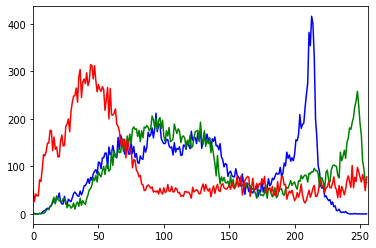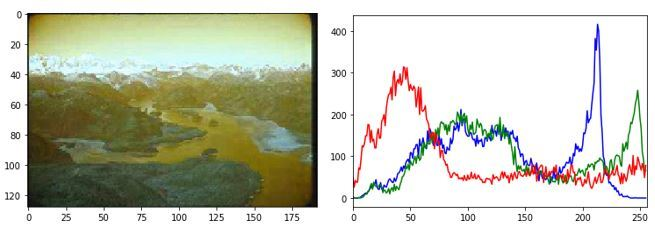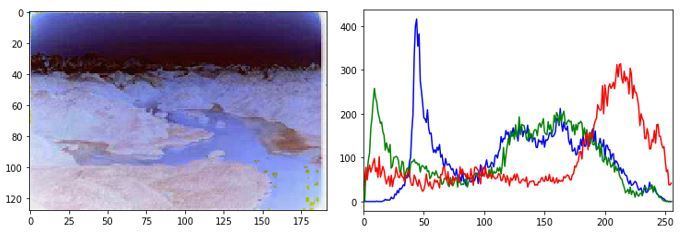Skip to content
Related Articles
Negative transformation of an image using Python and OpenCV
• Last Updated : 11 Mar, 2020

Image is also known as a set of pixels. When we store an image in computers or digitally, it’s corresponding pixel values are stored. So, when we read an image to a variable using OpenCV in Python, the variable stores the pixel values of the image. When we try to negatively transform an image, the brightest areas are transformed into the darkest and the darkest areas are transformed into the brightest.

As we know, a color image stores 3 different channels. They are red, green and blue. That’s why color images are also known as RGB images. So, if we need a negative transformation of an image then we need to invert these 3 channels.

Let’s see 3 channels of a color image by plotting it in the histogram.

Input Image –`# We need cv2 module for image ``# reading and matplotlib module``# for plotting``import` `cv2``import` `matplotlib.pyplot as plt``  ` `img_bgr ``=` `cv2.imread(``'scenary.jpg'``, ``1``)``  ` `color ``=` `(``'b'``, ``'g'``, ``'r'``)`` ` `for` `i, col ``in` `enumerate``(color):``    ``histr ``=` `cv2.calcHist([img_bgr], [i], ``None``, [``256``], [``0``, ``256``])``    ``plt.plot(histr, color ``=` `col)``    ``plt.xlim([``0``, ``256``])``     ` `plt.show()`

Output:Here, the 3 channels (Red, Green, Blue) are overlapped and create a single histogram. If you have studied about pixels and RGB before, you may know that each color contains 256 values. If RGB value of a color is (255, 255, 255) then that color is shown as white and If RGB value of a color is (0, 0, 0) then that color is shown as black. Like that, the above 3 channels also contain 256 numbers of pixels.

So, X-axis shows a total of 256 values (0 – 255) and Y-axis shows the total frequencies of each channel. As you can see in the histogram, the blue channel has the highest frequency and you can easily mark the amount of blue color present in the image by looking at it.

## Negative transformation of the image

Let’s create a negative transformation of the image. There 2 different ways to transform an image to negative using the OpenCV module. The first method explains negative transformation step by step and the second method explains negative transformation of an image in single line.

First method: Steps for negative transformation

1. Read an image
2. Get height and width of the image
3. Each pixel contains 3 channels. So, take a pixel value and collect 3 channels in 3 different variables.
4. Negate 3 pixels values from 255 and store them again in pixel used before.
5. Do it for all pixel values present in image.

Python code for 1st method: –

 `import` `cv2``import` `matplotlib.pyplot as plt`` ` ` ` `# Read an image``img_bgr ``=` `cv2.imread(``'scenary.jpg'``, ``1``)``plt.imshow(img_bgr)``plt.show()`` ` `# Histogram plotting of the image``color ``=` `(``'b'``, ``'g'``, ``'r'``)`` ` `for` `i, col ``in` `enumerate``(color):``     ` `    ``histr ``=` `cv2.calcHist([img_bgr], ``                         ``[i], ``None``,``                         ``[``256``], ``                         ``[``0``, ``256``])``     ` `    ``plt.plot(histr, color ``=` `col)``     ` `    ``# Limit X - axis to 256``    ``plt.xlim([``0``, ``256``])``     ` `plt.show()`` ` `# get height and width of the image``height, width, _ ``=` `img_bgr.shape`` ` `for` `i ``in` `range``(``0``, height ``-` `1``):``    ``for` `j ``in` `range``(``0``, width ``-` `1``):``         ` `        ``# Get the pixel value``        ``pixel ``=` `img_bgr[i, j]``         ` `        ``# Negate each channel by ``        ``# subtracting it from 255``         ` `        ``# 1st index contains red pixel``        ``pixel[``0``] ``=` `255` `-` `pixel[``0``]``         ` `        ``# 2nd index contains green pixel``        ``pixel[``1``] ``=` `255` `-` `pixel[``1``]``         ` `        ``# 3rd index contains blue pixel``        ``pixel[``2``] ``=` `255` `-` `pixel[``2``]``         ` `        ``# Store new values in the pixel``        ``img_bgr[i, j] ``=` `pixel`` ` `# Display the negative transformed image``plt.imshow(img_bgr)``plt.show()`` ` `# Histogram plotting of the``# negative transformed image``color ``=` `(``'b'``, ``'g'``, ``'r'``)`` ` `for` `i, col ``in` `enumerate``(color):``     ` `    ``histr ``=` `cv2.calcHist([img_bgr], ``                         ``[i], ``None``,``                         ``[``256``],``                         ``[``0``, ``256``])``     ` `    ``plt.plot(histr, color ``=` `col)``    ``plt.xlim([``0``, ``256``])``     ` `plt.show()`

Output :(Original image and it’s histogram)(Negative image and it’s histogram)

2nd method: Steps for negative transformation

1. Read an image and store it in a variable.
2. Subtract the variable from 1 and store the value in another variable.
3. All done. You successfully done the negative transformation.

Python code for 2nd method: –

 `import` `cv2``import` `matplotlib.pyplot as plt`` ` ` ` `# Read an image``img_bgr ``=` `cv2.imread(``'scenary.jpg'``, ``1``)`` ` `plt.imshow(img_bgr)``plt.show()`` ` `# Histogram plotting of original image``color ``=` `(``'b'``, ``'g'``, ``'r'``)`` ` `for` `i, col ``in` `enumerate``(color):``     ` `    ``histr ``=` `cv2.calcHist([img_bgr],``                         ``[i], ``None``,``                         ``[``256``],``                         ``[``0``, ``256``])``     ` `    ``plt.plot(histr, color ``=` `col)``     ` `    ``# Limit X - axis to 256``    ``plt.xlim([``0``, ``256``])``     ` `plt.show()`` ` `# Negate the original image``img_neg ``=` `1` `-` `img_bgr`` ` `plt.imshow(img_neg)``plt.show()`` ` `# Histogram plotting of``# negative transformed image``color ``=` `(``'b'``, ``'g'``, ``'r'``)`` ` `for` `i, col ``in` `enumerate``(color):``     ` `    ``histr ``=` `cv2.calcHist([img_neg],``                         ``[i], ``None``, ``                         ``[``256``],``                         ``[``0``, ``256``])``     ` `    ``plt.plot(histr, color ``=` `col)``    ``plt.xlim([``0``, ``256``])``     ` `plt.show()`

Output :(Original image and it’s histogram)(Negative image and it’s histogram)

Attention geek! Strengthen your foundations with the Python Programming Foundation Course and learn the basics.

To begin with, your interview preparations Enhance your Data Structures concepts with the Python DS Course. And to begin with your Machine Learning Journey, join the Machine Learning – Basic Level Course

My Personal Notes arrow_drop_up